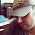## Monday, August 20, 2007

### Three KEAM 2007 Multiple Choice Questions from Heat and Thermodynamics

Four questions from Heat and Thermodynamics appeared in the Kerala Engineering Entrance 2007 question paper. One question was quite simple, meant for testing your knowledge of the temperature at which water has minimum volume, which most of you know, is 4º C. Here are the remaining three questions:
(1) A closed gas cylinder is divided into two parts by a piston held tight. The pressure and volume of gas in two parts respectively are (P, 5V) and (10 P, V). If now the piston is left free and the system undergoes isothermal process, then the volume of the gas in two parts respectively are
(a) 2 V, 4 V (b) 3 V, 3 V (c) 5 V, V (d) 4 V, 2 V (e) 2.5 V, 3.5 V
Since the temperature is constant (isothermal process), we can apply Boyle’s law. The piston will move towards the lower pressure side until the pressures on the two sides are the same. If this common pressure is P’, we have
P×5V + 10P×V = P’×6V, since the total volume is 6 V.
From this, P’ = (15/6) P.
Now, applying Boyle’s law to the gas on the two sides separarely, we have
P×5V = (15/6) P×V1 and
10P×V = (15/6) P×V2
where V1 and V2 are the volumes of the gas in the two parts respectively.
From the above equations, we obtain V1 = 2V and V2 = 4V [Option (a)].
(2) A Carnot engine with sink’s temperature at 17º C has 50% efficiency. By how much should its source temperature be changed to increase its efficiency to 60%?
(a) 225 K (b) 128º C (c) 580 K (d) 145 K (e) 145º C
We have efficiency η = (T1 T2)/T1 where T1 and T2 are the temperatures of the source and sink respectively.
Here, η = 0.5 and T2 = 17º C = 290 K. On substituting these values, we obtain
1– (290/T1) = 0.5, from which T1 = 580 K.
When the efficiency is 60%, we have
1– (290/T1') = 0.6, from which T1' = 725 K.
Therefore, the change in source temperature = 725 – 580 = 145 K.
(3) Two moles of oxygen is mixed with eight moles of helium. The effective specific heat of the mixture at constant volume is
(a) 1.3 R (b) 1.4 R (c) 1.7 R (d) 1.9 R (e) 1.2 R
Oxygen is diatomic and hence its molar specific heat at constant volume is (5/2) R (corresponding to 5 degrees of freedom).
Helium is mono-atomic and hence its molar specific heat at constant volume is (3/2) R (corresponding to 3 degrees of freedom).
Therefore, the total quantity of heat required to raise the temperature of two moles of oxygen and eight moles of helium through 1 K is
2×(5/2)R + 8×(3/2)R = 17 R.
Since the number of moles in the mixture is 10, the effective molar specific heat of the mixture is (17/10)R = 1.7 R.

1.2.